# GSEB Solutions Class 9 Maths Chapter 7 Triangles Ex 7.2

Gujarat Board GSEB Solutions Class 9 Maths Chapter 7 Triangles Ex 7.2 Textbook Questions and Answers.

## Gujarat Board Textbook Solutions Class 9 Maths Chapter 7 Triangles Ex 7.2

Question 1.
In an isosceles triangle ABC, with AB = AC, the bisectors of ∠B and ∠C intersect each other at O. Join A to O. Show that.
1. OB = OC
2. AO bisects ∠A
Solution:
1. AB = AC (given)
∠B = ∠C
(Angle opposite to equal sides are equal)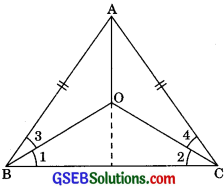∠B = ∠C (Dividing by 2)
(OB and OC angle bisectors of ∠B and ∠C)
⇒ ∠1 = ∠2
Hence OB = OC
(Sides opposite to equal angles are equal)

2. Now in ΔAOB and ΔAOC
AB = AC (given)
∠B = ∠C (Angle opposite to equal sides are equal)
⇒$$\frac {1}{2}$$∠B = $$\frac {1}{2}$$∠C (Dividing by 2)
⇒∠3 = ∠4
and OB = OC (Proved above)
Hence ∠AOB ≅ ∠AOC (by SAS congruency)
∴ ∠OAB = ∠OAC (By CPCT)
Hence AO bisects ∠A.Question 2.
In ΔABC, AD is the perpendicular bisector of BC (see in figure). Show that ΔABC is an isosceles triangle in which AB = AC.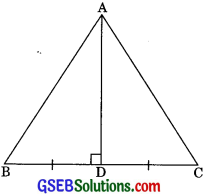Solution:
Given: ΔABC in which AD ⊥ BC
and BD = CD
To Prove: ΔABC is an isosceles triangle in which
AB = AC
BD = CD (AD is lar bisector of BC)
∴ AB = AC (by CPCT)
Therefore ABC is an isosceles triangle in which AB = AC.

Question 3.
ABC is an isosceles triangle in which altitudes BF and CF are drawn to equal sides AC and AB respectively (See figure). Show that these altitudes are equal.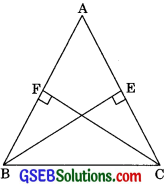Solution:
Given: ABC is an isosceles triangle in which BE and CF are altitudes on AC and AB respectively.
To Prove:
BE = CF
Proof: In ΔABE and ΔACF,
AB = AC (Equal sides of an isosceles triangle)
∠AEB = ∠AFC (each 90°)
∠BAE = ∠CAF (Common)
∴ ΔAEB ≅ ΔACF (By AAS congruency)
Hence BE = CF (by CPCT)Question 4.
ABC is a triangle in which altitudes BE and CF to sides AC and AB are equal (see figure). Show that
(i) ΔABE ≅ ΔACF
(ii) AB = AC (i.e. ABC is an isosceles triangle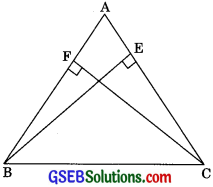Solution:
Given: ΔABC in which BE = CF.
(i) In ΔABE and ΔACF,
∠BAE = ∠CAF (Common)
∠AEB = ∠AFC (Each 900)
BE = CF (given)
∴ ΔABE ≅ ΔACF (By AAS congruency)

(ii) ΔABE ≅ ΔACF (Proved above in part i)
∴ AB = AC (by CPCT)
ΔABC is an isosceles triangle.

Question 5.
ABC and DBC are two isosceles triangles on the same base BC (see figure). Show that ∠ABD = ∠ACD.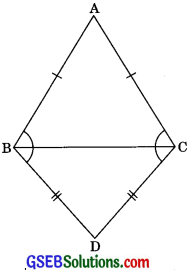Solution:
ABC is an isosceles triangle.
AB = AC (given)
∠ABC = ∠ACB ……..(1) (Angles opposite to equal sides are equal)
Now DBC is an isosceles triangle.
BD = CD (given)
∴ ∠DBC = ∠DCB (Angles opposite to equal sides are equal)
Adding equation (1) and (2), we get
∠ABC + ∠DBC = ∠ACB + ∠DCB
∠ABD = ∠ACDQuestion 6.
ΔABC is an isosceles triangle in which AB = AC. Side BA is produced to D such that AD = AB. (see figure). Show that ∠BCD is a right angle.
Or
In the given figure AB = AC and AB = AD, Prove that ∠BCD = 90°.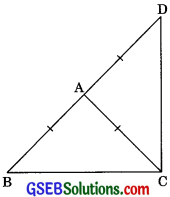Solution:
ΔABC is an isosceles triangle.
AB = AC (given)
∴ ∠ABC = ∠ACB
(Angles opposite to equal sides are equal)
In ΔACD,
But AB = AC
(Angles opposite to equal sides are equal)
Adding eqn. (1) and (2), we get
∠ABC + ∠ADC = ∠ACB + ∠ACD
∠ABC + ∠BDC = ∠BCD ………(3)
Now in ∠ABC,
∠ABC + ∠BDC + ∠BCD = 180° (By Angle Sum Property)
∠BCD + ∠BCD = 180°
[From eqn. (3)]
2 ∠BCD = 180°
∠BCD = $$\frac {180°}{2}$$
∠BCD = 90°Question 7.
ABC is a right-angled triangle in which ∠A = 90°, and AB = AC. Find ∠B and ∠C.
Solution:
In ΔABC,
AB = AC
∠B = ∠C
(Angles opposite to equal sides are equal)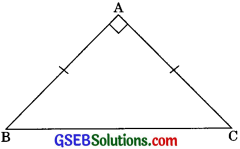Now in ∠ABC,
∠A + ∠B + ∠C = 180° (by angle sum property)
90°+ ∠C + ∠C = 180° (∠B = ∠C)
2∠C = 180° – 90°
2∠C = 90°
∠C = 45°
∠B = ∠C = 45°

Question 8.
Show that the angles of an equilateral triangle are 60° each.
Solution:
Given: ABC is an equilateral triangle.
To Show:
∠A = ∠B = ∠C = 60°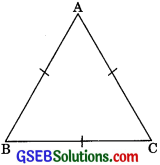Proof: ABC is an equilateral triangle.
∴ AB = AC
∴ ∠C = ∠B …….(1) (Angles opposite to equal sides are equal)
BC = AC
∠A = ∠B ………(2) (Angles opposite to equal sides are equal)
From eqn. (1) and (2), we get
∠A = ∠B = ∠C = x (Say)
Now in ΔABC,
∠A + ∠B + ∠C = 180°
x + x + x = 180°
3x = 180°
x = 60°
Hence ∠A + ∠B = ∠C = 60°